# Converting units of time

Here you will learn about converting units of time, including converting between seconds, minutes and hours.

Students will first learn about converting units of time as part of measurement and data in the 5 th grade, and expand on their understanding in ratios and proportional relationships in 6 th grade.

## What is converting units of time?

Converting units of time is converting between seconds, minutes and hours. To convert units of measurement, you need to first understand the relationships between the units and use the correct time conversion factor.

The most basic units of time are seconds, minutes, hours and days.

In the United States, the customary units of time is the system of measurements used. These measurements are used on a daily basis. Look at the conversion chart below:

\hspace{2.2cm} Customary Units of Time

1 year 365 days* / 12 months / 52 weeks
1 week 7 days
1 day 24 hours
1 hour 60 minutes
1 minute 60 seconds

*Note: In a leap year, an extra day is added to the calendar and the number of days in a year are 366. *

## Converting units of time using conversion tables

A conversion table is a table of equivalents that is used to change units of measure into other units. These are sometimes referred to as input-output tables.

You can use a conversion table to find the number of seconds in a certain number of minutes. See the table below:

MinuteSeconds
1 60
2 120
3 180

For every 60 seconds, another minute is added. If you wanted to find the number of seconds in 4 minutes, you could add another row to the conversion table and add 60 seconds to 180.

180+60=240

MinuteSeconds
1 60
2 120
3 180
4 \color{#189AB4}{240}

## Converting units of time using conversion factors

A conversion factor is the number used to change one set of units to another by either multiplying or dividing. Conversion factors are best used when dealing with a larger number of units.

When converting a larger number of seconds to minutes, it may be easier to use the conversion factor and divide the number of seconds by 60.

For example,

Convert 1,920 seconds to minutes.

1,920 \div 60=32 \text { minutes }

### What is converting units of time?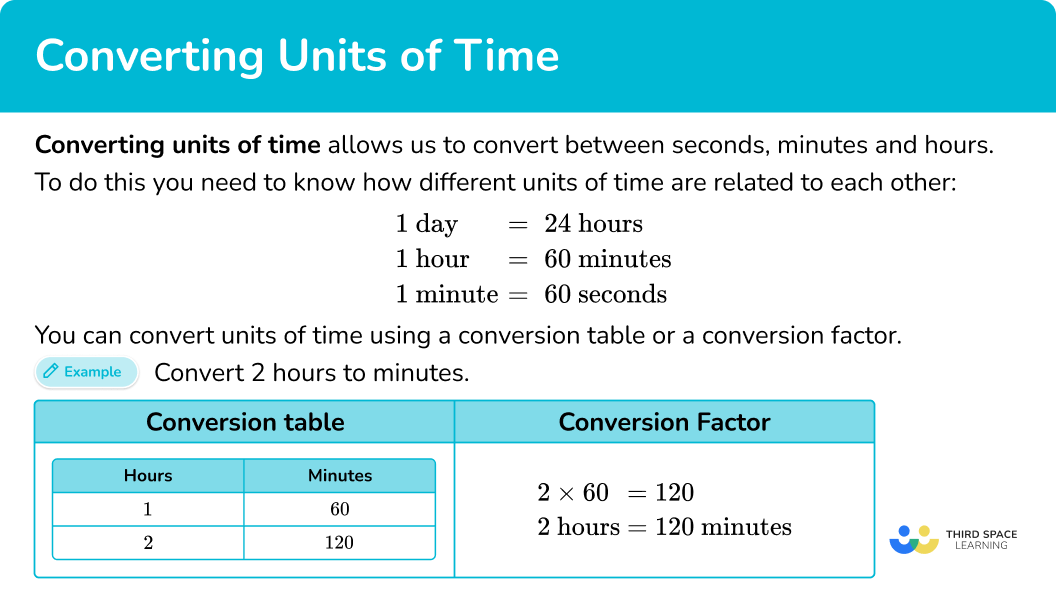## Common Core State Standards

How does this relate to 5 th grade math and 6th grade math?

• Grade 5: Measurement and Data (5.MD.A.1)
Convert among different-sized standard measurement units within a given measurement system (for example, convert 5 \, cm to 0.05 \, m ), and use these conversions in solving multi-step, real world problems.

• Grade 6: Ratios and Proportional Relationships (6.RP.A.3d)
Use ratio reasoning to convert measurement units; manipulate and transform units appropriately when multiplying or dividing quantities.

## How to convert units of time using conversion table

In order to convert units of time, you need to:

1. Check the units involved.
2. Draw conversion table and label with correct units.
3. Add on the number of units until you reach the final answer.

## Converting units of time examples

### Example 1: convert days to hours using conversion table

How many hours are there in 4 days?

1. Check the units involved.

1 \text { day }=24 \text { hours }

2Draw conversion table and label with correct units.

3Add on the number of units until you reach the final answer.

2 \text { days }=24+24=48 \text { hours }

3 \text { days }=48+24=72 \text { hours }

4 \text { days }=72+24=96 \text { hours }

There are 96 hours in 4 days.

### Example 2: convert hours to minutes using conversion table

How many minutes are there in 6 hours?

1 \text { hour }=60 \text { minutes. }

2 \text { hours }=60+60=120 \text { minutes }

3 \text { hours }=120+60=180 \text { minutes }

4 \text { hours }=180+60=240 \text { minutes }

5 \text { hours }=240+60=300 \text { minutes }

6 \text { hours }=300+60=360 \text { minutes }

There are 360 minutes in 6 hours.

## How to convert units of time using conversion factor

In order to convert units of time, you need to:

1. Check the units involved.
2. Multiply or divide by the conversion factor.

### Example 3: convert hours to hours and minutes

Find the number of hours and minutes in 1.4 hours.

1 \text { hour }=60 \text { minutes. }

So the conversion factor is 60.

Based on the decimal, we already know that there is 1 hour. Concentrate on the decimal part of the original number, 0.4. This part represents the minutes.

Multiply 0.4 by 60 to convert to minutes:

0.4 \times 60=24

1.4 hours is equal to 1 hour 24 minutes.

### Example 4: convert minutes to hours

Convert 324 minutes to hours.

1 \text { hour }=60 \text { minutes. }

So the conversion factor is 60.

You are going from a smaller unit (minutes) to a larger unit (hours), so you will divide the number of minutes by 60.

324 \div 60=5.4

324 minutes is 5.4 hours.

### Example 5: convert minutes to seconds

Convert 520 minutes to seconds.

1 \text { minute } = 60 \text { seconds. }

So the conversion factor is 60.

You are going from a larger unit (minutes) to a smaller unit (seconds), so you will multiply the number of minutes by 60.

520 \times 60=31,200

520 minutes is equal to 31,200 seconds.

### Example 6: converting units of time word problem

How many seconds are equal to 3 hours?

1 \text { hour } = 60 \text { minutes. }

1 \text { minute } = 60 \text { seconds. }

You will use the conversion factor of 60 two times.

First, you will need to find the number of minutes in 3 hours.

3 \times 60=180 \text { minutes }

You can now find the number of seconds in 180 minutes.

180 \times 60=10,800 \text { seconds }

There are 10,800 seconds in 3 hours.

### Teaching tips for converting units of time

• Students should be familiar with, or have access to, the important relationships within the different units of time. These can be displayed in the classroom or be included in a student’s interactive math notebook for easy reference.

• Time worksheets should only be used for students who are showing difficulty with telling time.

### Easy mistakes to make

• Base \bf{10} and base \bf{60}
A common misconception is to confuse the units used in the metric system with the units used for time.

The metric system uses base 10.
Each successive unit is 10 times larger than the previous one.
For example,

Time (for conversions between seconds, minutes and hours) uses base 60.
For example,

### Practice converting units of time questions

1) How many hours are there in 2 days? Use a conversion table to find the answer.

48 hours24 hours72 hours63 hoursFirst check the units involved in the problem.

1 \text { day } = 24 \text { hours. }

Draw a conversion chart and label with correct units.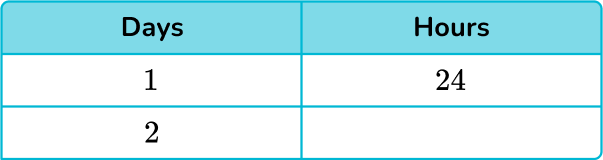Add on the number of units until you reach the final answer.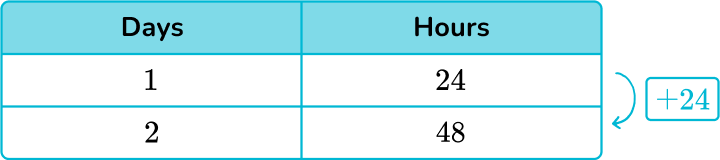24+24=48 \text { hours }

There are 48 hours in 2 days.

2) How many minutes are there in 3 hours? Use a conversion table to find the answer.

31 minutes190 minutes180 minutes310 minutesFirst check the units involved in the problem.

1 \text { hour } = 60 \text { minutes. }

Draw a conversion chart and label with correct units.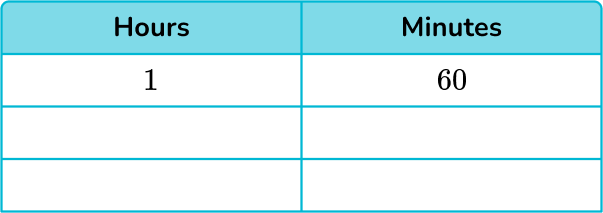Add on the number of units until you reach the final answer.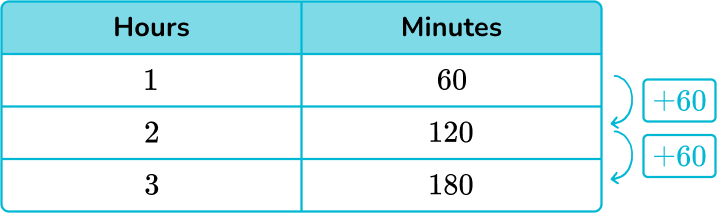60+60+60=180 \text { minutes }

There are 180 minutes in 3 hours.

3) Convert 4.35 hours to hours and minutes?

4 hours and 35 minutes4 hours and 25 minutes4 hours and 21 minutes4 hours and 28 minutesConcentrate on the decimal fraction part, which represents the minutes.

0.35\times 60=21

So 4.35 hours is 4 hours and 21 minutes.

4) Convert 75 minutes to hours.

0.75 hours1.5 hours minutes7.5 hours1.25 hoursThere are 60 minutes in an hour, so our conversion factor is 60.

Because you are going from a smaller unit to a larger unit, you will divide the number of minutes by 60.

75\div 60=1.25

75 minutes is the same as 1.25 hours.

5) Convert 135 minutes to hours and minutes.

13 hours and 5 minutes1 hours and 35 minutes2 hours and 25 minutes2 hours and 15 minutesThere are 60 minutes in an hour, so our conversion factor is 60.

Because you are going from a smaller unit to a larger unit, you will divide the number of minutes by 60.

135\div 60=2.25

There are 2 hours.

You will then focus on the decimal to find the number of minutes.

0.25\times 60=15

There are 15 minutes.

135 minutes is the same as 2 hours and 15 minutes.

6) How many hours are in 2 weeks?

336 hours48 hours302 hours225 hoursFirst, you will need to find the number of days in 2 weeks. There are 7 days in a week, so 7 \times 2=14 \text { days. }

There are 24 hours in 1 day, so multiply 14 by 24.

14 \times 24=336 \text { hours }

There are 336 hours in 2 weeks.

## Converting units of time FAQs

What is the smallest unit of time?

The second is the most commonly used and recognized smallest unit of time. However, in fields such as computing and electronics, the millisecond (\cfrac{1}{1,000 \, th} of a second ) and microsecond (\cfrac{1}{1,000,000 \, th} of a second) are also used.

How do you read an analog clock?

First, look at the hour hand (the shortest hand) to determine the hour. Then look at the minute hand (the longest hand) to determine the minutes. The minute hand points to the number of minutes past the hour.

How many days are in a month?

The number of days in a month varies depending on the month and the year. The following months have 31 days: January, March, May, July, August, October and December. The following months have 30 days: April, June, September, and November. The month of February has 28 days, except during a leap year, where it has 29 days.

## Still stuck?

At Third Space Learning, we specialize in helping teachers and school leaders to provide personalized math support for more of their students through high-quality, online one-on-one math tutoring delivered by subject experts.

Each week, our tutors support thousands of students who are at risk of not meeting their grade-level expectations, and help accelerate their progress and boost their confidence.